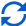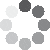Enter parameters
Enter parameters
LENGTH, WIDTH & Thickness
Length:
ft
• inches (in)
• feet (ft)
• yards (yd)
• centimeters (cm)
• meters (m)
Width:
in
• inches (in)
• feet (ft)
• yards (yd)
• centimeters (cm)
• meters (m)
Thickness:
in
• inches (in)
• feet (ft)
• yards (yd)
• centimeters (cm)
• meters (m)
Number of boards:
Price per board foot:Result
Total board feet:
0
Total cost:
$(2 votes, average: 5.00 out of 5)Loading... Similar Calculators: Real-time graphics. Make the calculations and see the changes. Embed Similar Calculators:Contents: ## What is a board foot? A board foot is a common unit of measurement for hardwood lumber. In contrast to a square foot, which is an area, a board foot is a volume. It has the dimensions $$\text{1 ft (length) × 1 ft (width) × 1 in (thickness)}$$ Therefore, a single board foot has volume (in cubic inches) equal to $$\text{12 in × 12 in × 1 in = 144 in}^3$$ Or, equivalently, the volume in the units ft. in² is $$\text{1 ft × 12 in × 1 in = 12 ft. in}^2$$ Now: Boards are sold in all different sizes. How does the board foot calculator compute board footage in general? ## How is board footage calculated? It turns out that the answer is simpler than you think! First, estimate the total volume of a given board using the formula for the volume of a rectangular solid. $$\text{Volume of board = length (ft) × width (in) × thickness (in)}$$ Then the total number of board feet is given by dividing the volume of the board by 12: $$\text{Board feet in single board} = {\text{volume of board (ft. in}^2) \over 12}$$ If you have more than one board, then multiply the total number of board feet in a single board by the number of boards: $$\text{Total board feet = board feet in single board × number of boards}$$ Put it all together to arrive at a single board foot formula: $$\text{Total board feet} = {\text{length (ft) × width (in) × thickness (in)} \over 12} × \text{number of boards}$$ ## How is cost calculated? Once total board feet has been assessed, it is easy to find the cost: $$\text{Total cost = price per board foot × total board feet}$$ ## Step-by-step example For this example, suppose that you need 10 boards that are 2.5 feet long, 4.5 inches wide, and 2 inches thick. 1. Calculate the number of board feet in one of the boards:$$\text{Board feet in one board} = {2.5 × 4.5 × 2 \over 12} = \text{1.875 bd. ft.}$$ 2. Multiply by the total number of boards (10) to get the total number of board feet:$$\text{Total board feet = 1.875 × 10 = 18.75 bd. ft.}$$ 3. If you know the cost per board foot, then multiply the result by this number to get the total cost for the 10 boards. Let’s assume the cost for one board foot is$6.50.$$\text{Total cost = 6.50 × 18.75 = \121.88}$$
Embed this calculator on your site!Add live graphics
Copied to clipboard! Preview